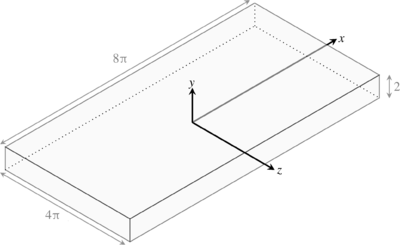# DNS 1-2 Description

(diff) ← Older revision | Latest revision (diff) | Newer revision → (diff)

# Introduction

The turbulent Channel Flow is one of the canonical flows used to study turbulence in wall bounded turbulence. DNS of turbulent channel flow were undertaken at $Re_{\tau }=180$. DNS were undertaken using PyFR (http://www.pyfr.org/) version 1.12.0:

• based on the high-order flux reconstruction method of Huynh
• compressible solver
• a Rusanov Riemann solver was employed to calculate the inter-element fluxes
• an explicit RK45[2R+] scheme was used to advance the solution in time
• Fifth order polynomials are used for the computations

# Review of previous studies

Turbulent channel has been one of the canonical test cases to study the characteristics of wall bounded turbulence. The simplified setup proposed by Kim et al. (1987) is now a standard test case for wall bounded turbulent flow simulations. The setup is computationally inexpensive as it a periodic channel flow with periodic boundary conditions in the spanwise and streamwise directions. With recent progress made in computing infrastructure, Reynolds numbers as large as $Re_{\tau }=4000$(Bernardini et al. 2014) and $Re_{\tau }=6000$(Pirrozoli et al. 2021) are now available. Nevertheless, the $Re_{\tau }=180$case by Kim et al. still remains as a benchmark case for wall bounded turbulent flow simulations.

# Description of the test case

An idealised channel flow, without side walls, is considered. The details of the case are given in Iyer et al.(2019). The current set of simulations has a higher grid resolution in the wall normal direction where there are 174 solution points compared to 95 points in the simulations of Iyer et al.

## Geometry and flow parameters

The geometry is a cuboid of dimensions $8\pi$units in the streamwise direction $(x)$, 2 units in the transverse direction $(y)$and $4\pi$units in the spanwise direction $(z)$, as shown in figure 1. The dimensions are normalised by the channel half-width, $h$and centreline velocity.Figure 1: Computational domain from Iyer et al. (2019)

## Boundary conditions

Periodicity conditions are imposed in the streamwise and spanwise boundaries which gives a flow developing in time. The transverse boundaries are no-slip walls. The initial density and pressure fields are uniform. The initial velocity field is $(u,v,w)=\left(1-y^{2}/h^{2},0,0\right)$. The solution is started at order 2 and progressively increased to order 5.

References

1. Huynh, H. T., A flux reconstruction approach to high-order schemes including discontinuous Galerkin methods, AIAA, 2007-4079, 1-42, 2007.
2. Kennedy, C. A., Carpenter, M. H. & Lewis, R. M, Low-storage, explicit Runge–Kutta schemes for the compressible Navier–Stokes equations, Appl. Numer. Maths, 35(3), 177-219, 2000.
3. Kim,J., Moin, P. & Moser, R., Turbulence statistics in fully developed channel flow at low Reynolds number, J. Fluid Mech. 177, 133-166, 1987
4. A. Iyer, F. D. Witherden, S. I. Chernyshenko & P. E. Vincent, Identifying eigenmodes of averaged small-amplitude perturbations to turbulent channel flow, Journal of Fluid Mechanics, 875 (758-780), 2019

Contributed by: Arun Soman Pillai, Lionel Agostini, Peter Vincent — Imperial College London